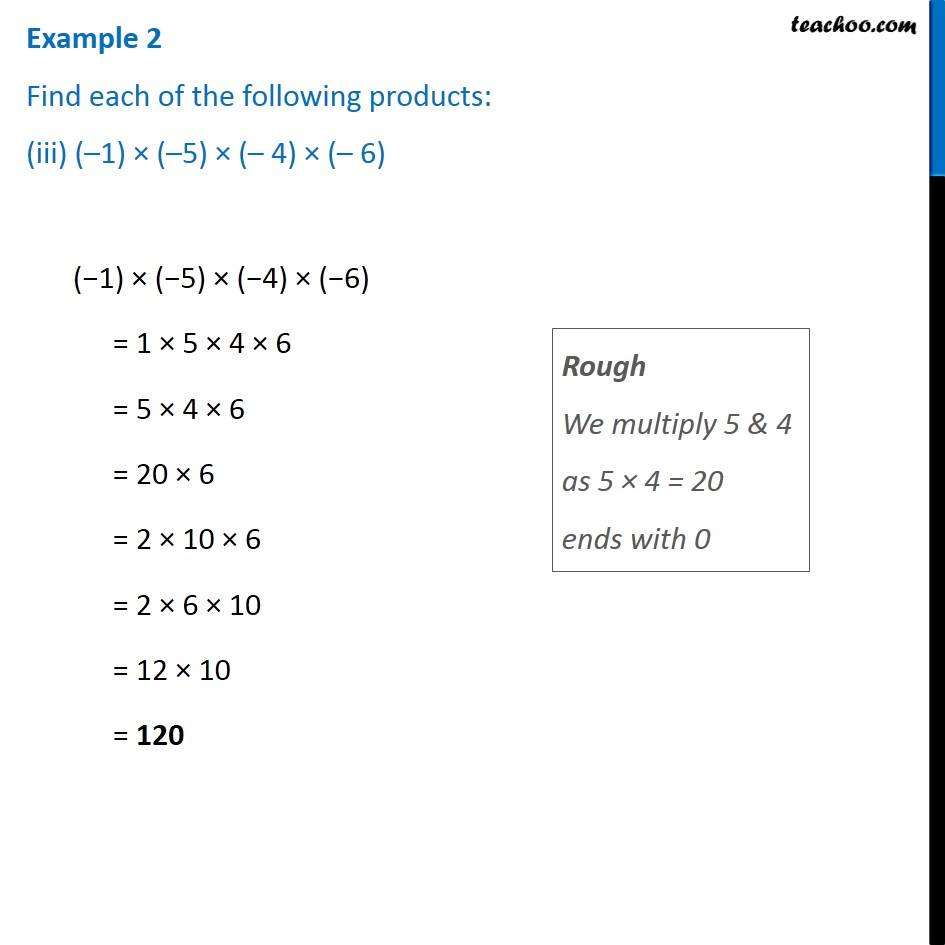1. Chapter 1 Class 7 Integers
2. Serial order wise
3. Examples

Transcript

Example 2 Find each of the following products: (iii) ( 1) ( 5) ( 4) ( 6) Rough We multiply 5 & 4 as 5 4 = 20 ends with 0 ( 1) ( 5) ( 4) ( 6) = 1 5 4 6 = 5 4 6 = 20 6 = 2 10 6 = 2 6 10 = 12 10 = 120